# Related Videos

## Solve $\int x\cos\left(2x^2+3\right)dx$

Go!
1
2
3
4
5
6
7
8
9
0
a
b
c
d
f
g
m
n
u
v
w
x
y
z
.
(◻)
+
-
×
◻/◻
/
÷
2

e
π
ln
log
log
lim
d/dx
Dx
|◻|
=
>
<
>=
<=
sin
cos
tan
cot
sec
csc

asin
acos
atan
acot
asec
acsc

sinh
cosh
tanh
coth
sech
csch

asinh
acosh
atanh
acoth
asech
acsch

### Videos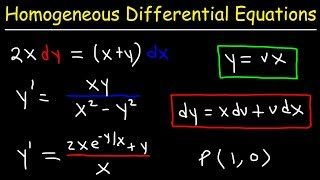### Homogeneous Differential Equations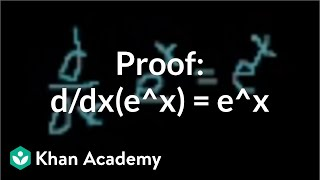### Proof: d/dx(e^x) = e^x | Taking derivatives | Differential Calculus | Khan Academy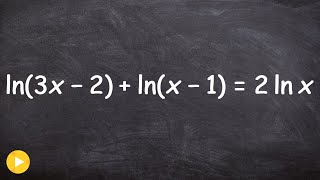### Pre-Calculus - How to solve a logarithmic equation with extraneous solution, ln(3x-2)+ln(x-1)=2ln(x)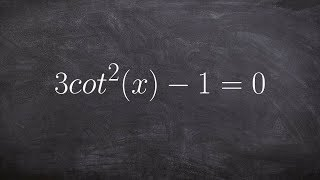### Pre-Calculus - Solving trigonometric equations ex 3, 3(cotx)^2 - 1 = 0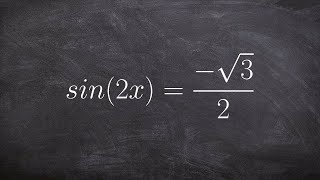### Pre-Calculus - Solving trigonometric equations with multiple angles ex 3, sin(2x) = -root(3)/2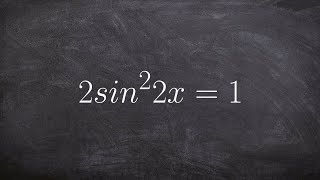### Pre-Calculus - Learn to solve multiple angles in trigonometry 2sin^2x=1

$\int\left(x\cdot\cos\left(2x^2+3\right)\right)dx$